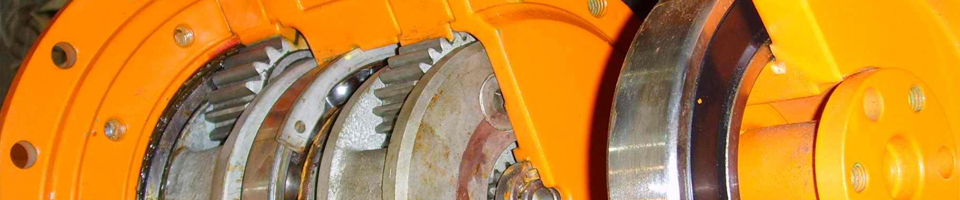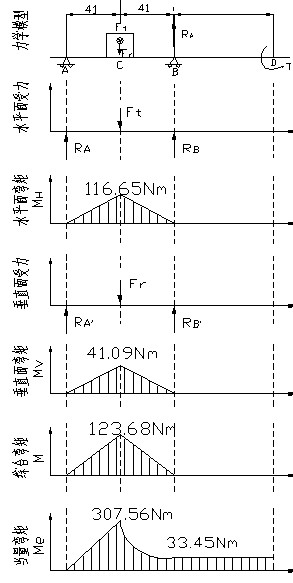圆柱齿轮减速机E-mail：28344966@qq.com

一级圆柱齿轮减速机输出轴的设计计算

2作用在齿轮上的转矩为：T1 =5.08×105N·mm  3求圆周力：FtFt=2T2/d2=2×5.08×105/270=3762.96N
4求径向力FrFr=Ft·tanα=3762.96×tan200=1369.61N Ft，Fr的方向如下图所示    （5）轴长支反力 根据轴承支反力的作用点以及轴承和齿轮在轴上的安装位置，建立力学模型。  水平面的支反力：RA=RB=Ft/2 = 1881.48 N  垂直面的支反力：由于选用深沟球轴承则Fa=0 那么RA’=RB’ =Fr×62/124= 684.81 N （6）画弯矩图  右起第四段剖面C处的弯矩：  水平面的弯矩：MC=RA×62= 116.65 Nm  垂直面的弯矩：MC1’= MC2’=RA’×62=41.09 Nm  合成弯矩：    （7）画转矩图： T= Ft×d2/2=508.0 Nm  （8）画当量弯矩图    因为是单向回转，转矩为脉动循环，α=0.6    可得右起第四段剖面C处的当量弯矩：
（9）判断危险截面并验算强度 1右起第四段剖面C处当量弯矩最大，而其直径与相邻段相差不大，所以剖面C为危险截面。 已知MeC2=307.56Nm ,由课本表13-1有: ［σ-1］=60Mpa   则： σe= MeC2/W= MeC2/(0.1·D43) =307.56×1000/(0.1×603)=14.24 Nm<［σ-1］ 2右起第一段D处虽仅受转矩但其直径较小，故该面也为危险截面：  σe= MD/W= MD/(0.1·D13) =304.8×1000/(0.1×453)=33.45 Nm<［σ-1］  所以确定的尺寸是安全的。    以上计算所需的图如下：[本文观点非特殊说明，均有泰兴减速机-圆柱齿轮减速机整理原创所得，（转载必究）如要转载请保留相应的源地址，尊重他人劳动成果]